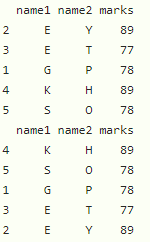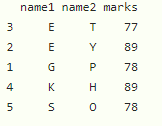Open in App
Not now

# How to Sort Values Alphabetically in R?

• Last Updated : 28 Nov, 2021

In this article, we will discuss how to sort values alphabetically in R Programming Language.

## Sorting vector Alphabetically

Here we are using sort() function to sort a vector alphabetically.

Syntax:

`sort(vector)`

where, vector is the input vector

Example:

## R

 `# create a vector``vector1 = ``c``(``'G'``, ``'E'``, ``'E'``, ``'K'``, ``'S'``)`` ` `# sort the vector``print``(``sort``(vector1))`

Output:

` "E" "E" "G" "K" "S"`

## Sorting Data Frame Column Alphabetically

We can create a dataframe by using date.frame() function. We can sort a dataframe column by using order() function

Syntax:

`dataframe[order(dataframe\$column_name),]`

where,

• dataframe is the input dataframe
• column_name is the column that includes alphabetical values based on this column

Example:

## R

 `# create a dataframe with 3 columns``data = ``data.frame``(name1=``c``(``'G'``, ``'E'``, ``'E'``, ``'K'``, ``'S'``),``                  ``name2=``c``(``'P'``, ``'Y'``, ``'T'``, ``'H'``, ``'O'``), ``                  ``marks=``c``(78, 89, 77, 89, 78))`` ` `# sort the dataframe based on name1 column``print``(data[``order``(data\$name1), ])`` ` `# sort the dataframe based on name2 column``print``(data[``order``(data\$name2), ])`

Output:## Method 3: Sort Multiple Columns Alphabetically

We can also sort multiple columns in dataframe by using order function.

Syntax:

dataframe[with(dataframe, order(column1, column2,.,column n)), ]

Example:

## R

 `# create a dataframe with 3 columns``data = ``data.frame``(name1=``c``(``'G'``, ``'E'``, ``'E'``, ``'K'``, ``'S'``), ``                  ``name2=``c``(``'P'``, ``'Y'``, ``'T'``, ``'H'``, ``'O'``), ``                  ``marks=``c``(78, 89, 77, 89, 78))`` ` `# sort the dataframe based on name1 and ``# name2 columns``print``(data[``with``(data, ``order``(name1, name2)), ])`

Output:My Personal Notes arrow_drop_up